# BE Biotechnology Semester 1 (FE First Year) - University of Mumbai Question Bank Solutions for Engineering Mechanics

Subjects
Topics
Subjects
Popular subjects
Topics
Engineering Mechanics
< prev  1 to 20 of 84  next >

Find the resultant of the force system as shown in the given figure.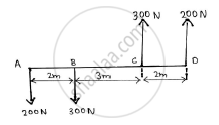System of Coplanar Forces
Chapter:  System of Coplanar Forces
Concept: Resultant of concurrent forces

Determine the position of the centroid of the plane lamina. Shaded portion is removed.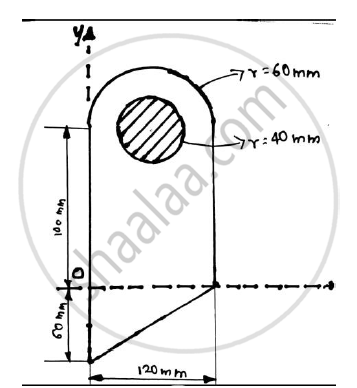Center of Gravity and Centroid for Plane Laminas
Chapter:  Center of Gravity and Centroid for Plane Laminas
Concept: Centroid for Plane Laminas

Explain the conditions for equilibrium of forces in space.

 Equilibrium of System of Coplanar Forces
Chapter:  Equilibrium of System of Coplanar Forces
Concept: Condition of equilibrium for concurrent forces

Referring to the truss shown in the figure. Find :
(a) Reaction at D and C
(b)Zero force members.
(c)Forces in member FE and DC by method of section.
(d)Forces in other members by method of joints.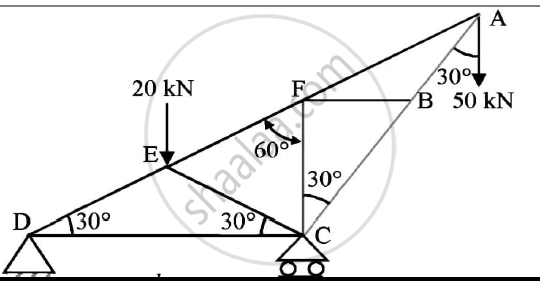Analysis of Plane Trusses
Chapter:  Analysis of Plane Trusses
Concept: Analysis of Plane Trusses by Using Method of Joints

Determine the force P required to move the block A of 5000 N weight up the inclined plane, coefficient of friction between all contact surfaces is 0.25. Neglect the weight of the wedge and the wedge angle is 15 degrees.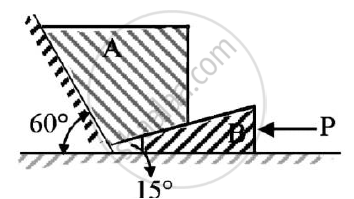Given : Weight of block A = 5000 N
μs=0.25
Wedge angle = 15º

To find : Force P required to move block A up the inclined plane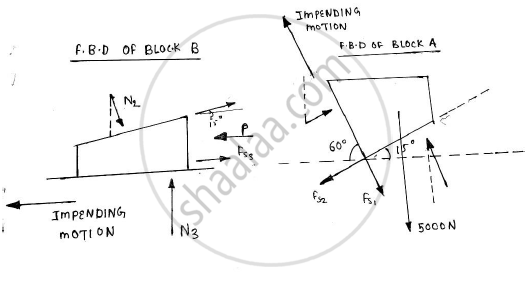Equilibrium of System of Coplanar Forces
Chapter:  Equilibrium of System of Coplanar Forces
Concept: Condition of equilibrium for concurrent forces

Determine the tension in a cable BC shown in fig by virtual work method.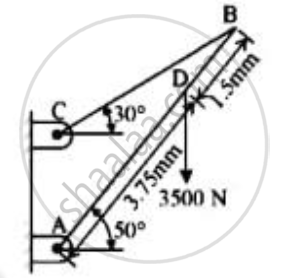Given: F=3500 N
ϴ = 50o
Length of rod = 3.75 mm + 1.5 mm = 5.25 mm
To find : Tension in cable BC

 Principle of Virtual Work
Chapter:  Principle of Virtual Work
Concept: Applications on Equilibrium Mechanisms

In the rocket arm shown in the figure the moment of ‘F’ about ‘O’ balances that P=250 N.Find F.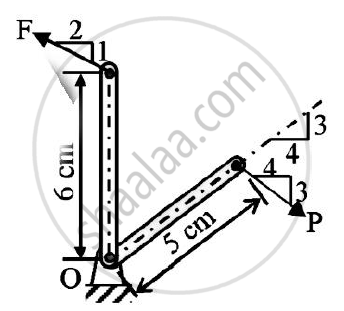System of Coplanar Forces
Chapter:  System of Coplanar Forces
Concept: Resultant of concurrent forces

Find the centroid of the shaded portion of the plate shown in the figure.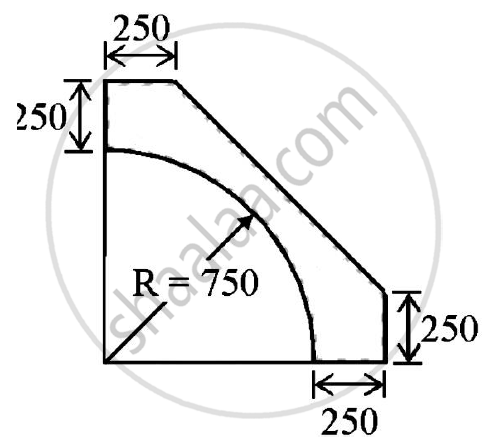Center of Gravity and Centroid for Plane Laminas
Chapter:  Center of Gravity and Centroid for Plane Laminas
Concept: Centroid for Plane Laminas

A 50 N collar slides without friction along a smooth and which is kept inclined at 60o to the horizontal.
The spring attached to the collar and the support C.The spring is unstretched when the roller is at A(AC is horizontal).
Determine the value of spring constant k given that the collar has a velocity of 2.5 m/s when it has moved 0.5 m along the rod as shown in the figure.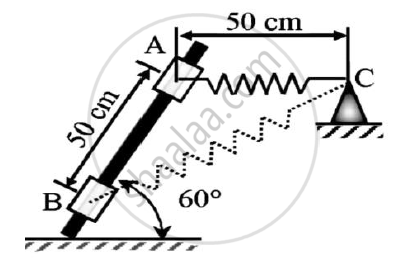[11.02] Work and Energy
Chapter: [11.02] Work and Energy
Concept: Principle of Work and Energy

Find the force F4 , so as to give the resultant of the force as shown in the figure given below.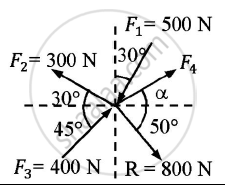Given : Forces and their resultant
To find : Force F4

 System of Coplanar Forces
Chapter:  System of Coplanar Forces
Concept: Resultant of concurrent forces

A particle starts from rest from origin and it’s acceleration is given by a = k/(x+4)^2     m⁄s^2.Knowing that v = 4 m/s when x = 8m,find :
(1)Value of k
(2)Position when v = 4.5 m/s

Given :  Particle starts from rest
a = k/(x+4)^2    m⁄s^2
v = 4m/s at x = 8m
To find : Value of k and position when v= 4.5m/s

[11.01] Force and Acceleration
Chapter: [11.01] Force and Acceleration
Concept: Introduction to Basic Concepts

Two cylinders 1 and 2 are connected by a rigid bar of negligible weight hinged to each cylinder and left to rest in equilibrium in the position shown under the application of force P applied at the center of cylinder 2.
Determine the magnitude of force P.If the weights of the cylinders 1 and 2 are 100N and 50 N respectively.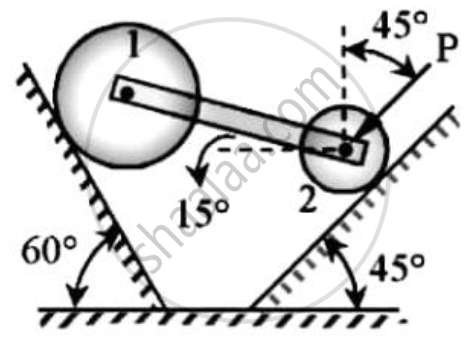Principle of Virtual Work
Chapter:  Principle of Virtual Work
Concept: Applications on Equilibrium Mechanisms

What is a zero force member in a truss? With examples state the conditions for a zero force member.

 Analysis of Plane Trusses
Chapter:  Analysis of Plane Trusses
Concept: Analysis of Plane Trusses by Using Method of Joints

In the truss shown in figure,compute the forces in each member.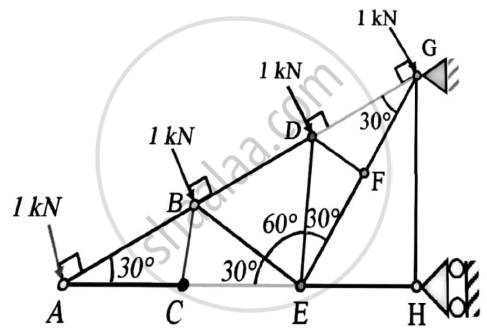Analysis of Plane Trusses
Chapter:  Analysis of Plane Trusses
Concept: Analysis of Plane Trusses by Using Method of Joints

The resultant of the three concurrent space forces at A is 𝑹̅ = (-788𝒋̅) N. Find the magnitude of F1,F2 and F3 forces.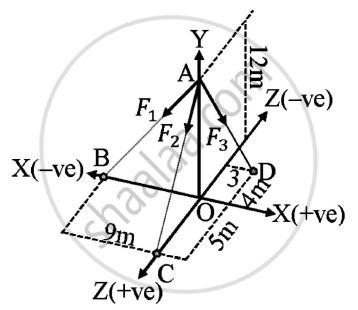Given : A=(0,12,0)
B=(-9,0,0)
C=(0,0,5)
D=(3,0,-4)
Resultant of forces = (-788𝑗̅) N
To find : Magnitude of forces F1,F2,F3

 System of Coplanar Forces
Chapter:  System of Coplanar Forces
Concept: Resultant of concurrent forces

Refer to figure.If the co-efficient of friction is 0.60 for all contact surfaces and θ = 30o,what force P applied to the block B acting down and parallel to the incline will start motion and what will be the tension in the cord parallel to inclined plane attached to A.
Take WA=120 N and WB=200 N.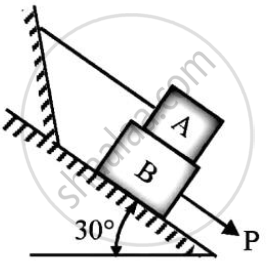Equilibrium of System of Coplanar Forces
Chapter:  Equilibrium of System of Coplanar Forces
Concept: Condition of equilibrium for concurrent forces

Determine the required stiffness k so that the uniform 7 kg bar AC is in equilibrium when θ=30o.
Due to the collar guide at B the spring remains vertical and is unstretched when θ = 0o.Use principle of virtual work.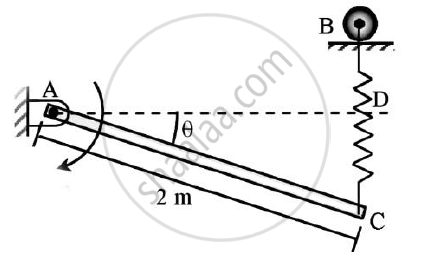Principle of Virtual Work
Chapter:  Principle of Virtual Work
Concept: Applications on Equilibrium Mechanisms

The system in figure is initially at rest.
Neglecting friction determine the force P required if the velocity of the collar is 5 m/s after 2 sec and corresponding tension in the cable.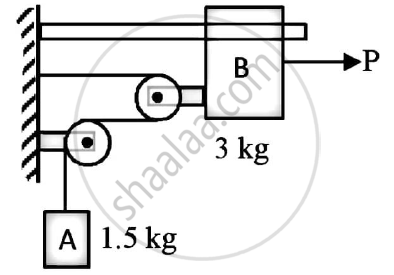Friction
Chapter:  Friction
Concept: Introduction to Laws of Friction

A truss is loaded and supported as shown.Determine the following:
(1)Identify the zero force members,if any
(2)Find the forces in members EF,ED and FC by method of joints.
(3)Find the forces in members GF,GC and BC by method of sections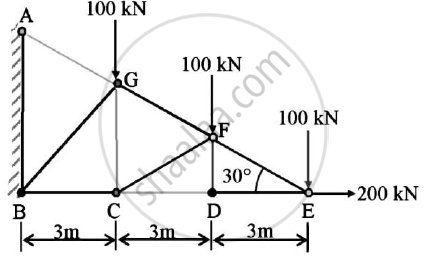Analysis of Plane Trusses
Chapter:  Analysis of Plane Trusses
Concept: Analysis of Plane Trusses by Using Method of Joints

b)A cylinder has a mass of 20kg and is released from rest when h=0 as shown in the figure.Determine its speed when h=3m.The springs have an unstretched length of 2 m.Take k=40 N/m.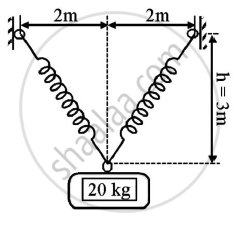Given : m=20 kg
h=0
k=40 N/m
To find : Speed when h=3m

[11.02] Work and Energy
Chapter: [11.02] Work and Energy
Concept: Principle of Work and Energy
< prev  1 to 20 of 84  next >